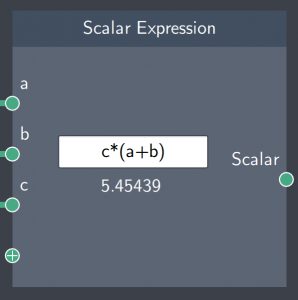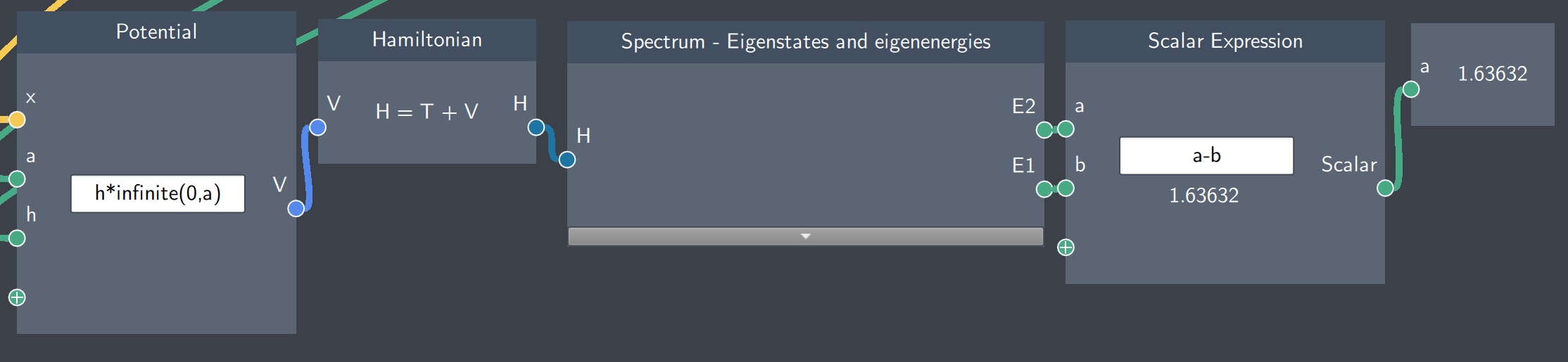# Scalar Expression

## Description

With this node, you can combine or modify scalar quantities and output any scalar resulting from the scalar arithmetic operation.## Inputs

The node has the following input:

• Scalars ($a$, $b$, $c$ etc.): Scalar quantities input from other nodes

## Content

In the content text-field, an arithmetic operation is defined to combine or modify the scalar quantities.

## Output

• Scalar ($a$): The scalar resulting from the arithmetic operation of the scalar inputs.

## Example

In the example below, the Scalar Expression node calculates the difference between the first two eigenstates of an infinite well. This tells us the energy spacing between the states of this system. Moreover, the energy spacing between any higher states can also be calculated, and the potential can be modified to calculate the energy spacing between states in other systems as well.